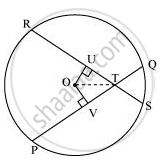# If Two Equal Chords of a Circle Intersect Within the Circle, Prove that the Line Joining the Point of Intersection to the Centre Makes Equal Angles with the Chords. - Mathematics

If two equal chords of a circle intersect within the circle, prove that the line joining the point of intersection to the centre makes equal angles with the chords.

#### SolutionLet PQ and RS are two equal chords of a given circle and they are intersecting each other at point T.

Draw perpendiculars OV and OU on these chords.

In ΔOVT and ΔOUT,

OV = OU (Equal chords of a circle are equidistant from the centre)

∠OVT = ∠OUT (Each 90°)

OT = OT (Common)

∴ ΔOVT ≅ ΔOUT (RHS congruence rule)

∴ ∠OTV = ∠OTU (By CPCT)

Therefore, it is proved that the line joining the point of intersection to the centre makes equal angles with the chords.

Concept: Equal Chords and Their Distances from the Centre
Is there an error in this question or solution?

#### APPEARS IN

NCERT Class 9 Maths
Chapter 10 Circles
Exercise 10.4 | Q 3 | Page 179